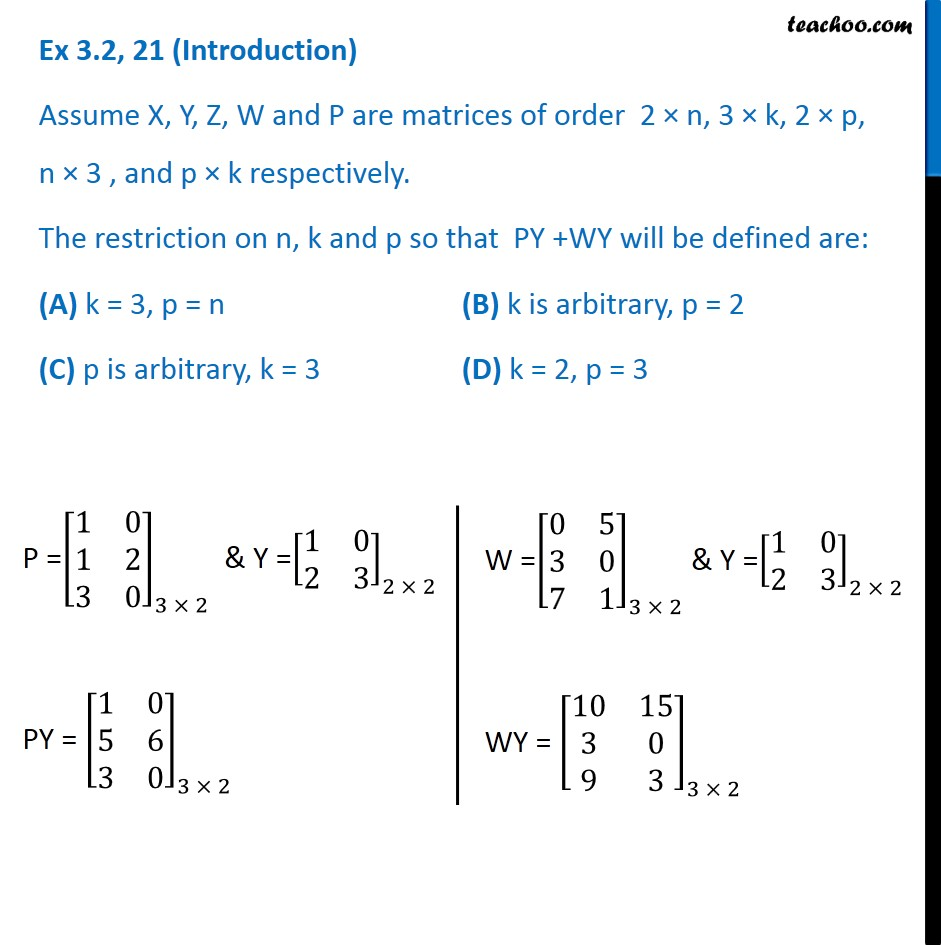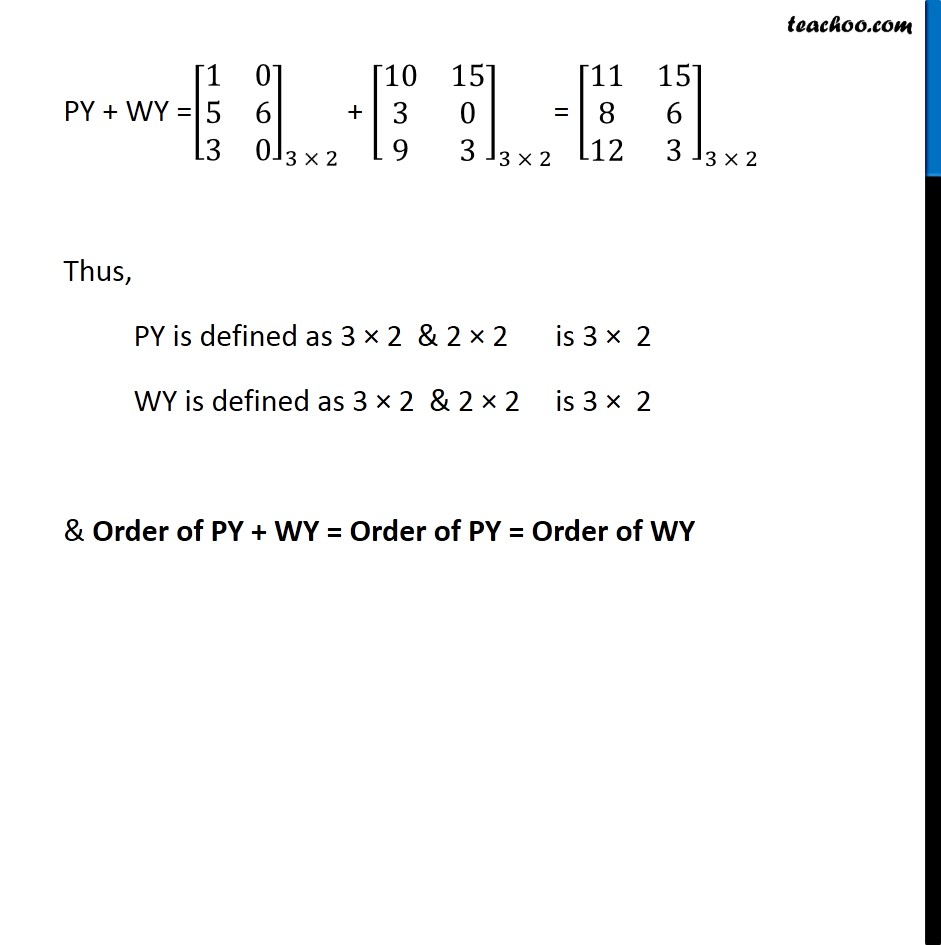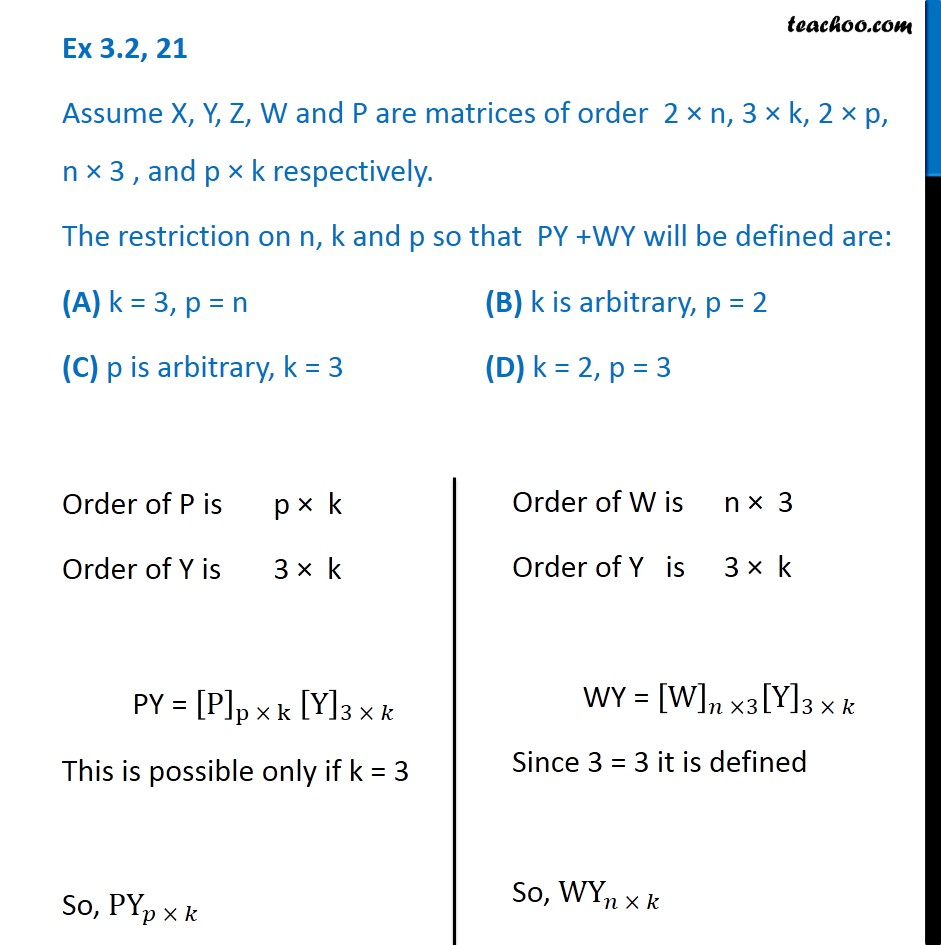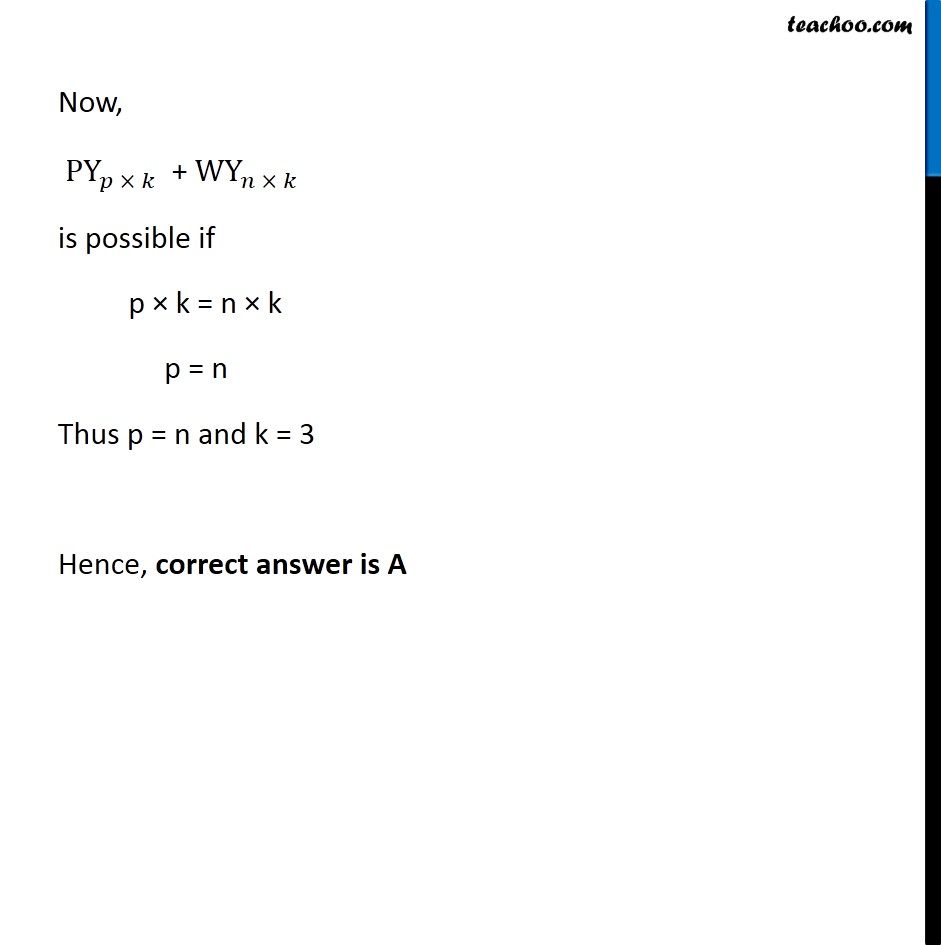Multiplication of matrices

Chapter 3 Class 12 Matrices
Concept wiseLearn in your speed, with individual attention - Teachoo Maths 1-on-1 Class

### Transcript

Ex 3.2, 21 (Introduction) Assume X, Y, Z, W and P are matrices of order 2 n, 3 k, 2 p, n 3 , and p k respectively. The restriction on n, k and p so that PY +WY will be defined are: (A) k = 3, p = n (B) k is arbitrary, p = 2 (C) p is arbitrary, k = 3 (D) k = 2, p = 3 Ex 3.2, 21 Assume X, Y, Z, W and P are matrices of order 2 n, 3 k, 2 p, n 3 , and p k respectively. The restriction on n, k and p so that PY +WY will be defined are: (A) k = 3, p = n (B) k is arbitrary, p = 2 (C) p is arbitrary, k = 3 (D) k = 2, p = 3 Order of P is p k Order of Y is 3 k PY = [P]_(p k) [Y]_(3 ) This is possible only if k = 3 So, PY_( ) Now, PY_( ) + WY_( ) is possible if p k = n k p = n Thus p = n and k = 3 Hence, correct answer is A